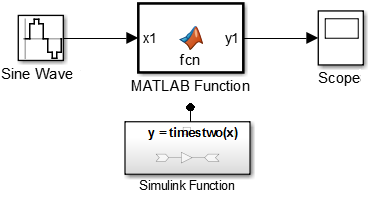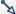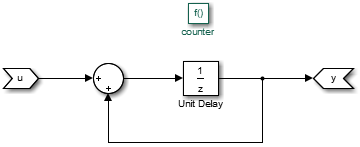## Simulink function callers: Function Caller block, MATLAB Function block, Stateflow chart

Simulink® functions have an interface with input and output arguments similar to programming languages. Simulink function callers send data through input arguments to Simulink functions, execute the function, and then receive data back from the function through output arguments. You can call a Simulink function using:

• Function Caller blocks

• MATLAB Function blocks

• Stateflow® charts

The following sections show how to call a Simulink function. The function ```y = timestwo(x)``` multiplies a value (`x`) from a caller by `2`, and then sends the calculated value (`y`) back to the caller. To create the functions, see Simulink functions: Simulink Function block, exported Stateflow graphical and MATLAB functions.

To open completed model with Simulink functions and function callers, see `ex_simulink_functions_and_function_callers`.

### Use a Function Caller block to call a Simulink Function block

Set up a Function Caller block to send data through an input argument to a Simulink Function block, and receive data back from the function through an output argument.

2. Open the Function Caller dialog box. In the Function prototype box, enter `y = timestwo(x)`. This function prototype creates an input port `x` and output port `y` on the Function Caller block.

Note

Typing in a blank text box displays a list of previously created function prototypes that match the text you are typing.

Note

The function and argument names for the Simulink Function block and the Function prototype for the Function Caller block must match exactly.#### Test the function call

1. Add a Sine Wave block to provide test data for the input and a Scope block to view results from the output.2. Run a simulation. The input sine wave with an amplitude of `2` is doubled.### Use a MATLAB Function block to call a Simulink Function block

Set up a MATLAB Function block to send data through an input argument to a Simulink Function block, and receive data back from the function through an output argument.

2. Double-click the block, which opens the MATLAB® editor. Enter the function call ```y1 = timestwo(x1)```.Note

The argument names for the function you define in the MATLAB Function block do not have to match the argument names for the function that you define with a Simulink Function block. For a Function Caller block that calls a Simulink Function block, argument names must match.

Note

MATLAB Function blocks only support discrete and fixed-in-minor sample times.#### Test the function call

1. Add a Sine Wave block to provide test data for the input and a Scope block to view results from the output.2. For the Sine Wave block, set the Sample time to `0.01`. For the model, open the Configuration Parameters dialog box to the solver pane. Set Type to `Fixed-step` and Fixed-step size to `0.01`.

3. Run a simulation.

### Use a Stateflow chart to call a Simulink Function block

Set up a Stateflow chart to send data through an input argument to a Simulink Function block, and receive data back from the function through an output argument.

1. Add a Stateflow Chart to your Simulink model. Double-click on the Simulink block diagram. In the search box, enter `chart`, and then from the search results, select `Chart`.

2. Double-click the chart to open it.

3. From the left-side toolbar, click and drag the default transition icononto the chart.

4. Add an input port to the chart. Open the Model Explorer. In the left pane, select `Chart`. From the menu, select Add > Data. Set Name to `x1` and Scope to `Input`.

Note

The argument names for the function you define in the Stateflow chart do not have to match the argument names for the function that you define with a Simulink Function block. For a Function Caller block that calls a Simulink Function block, argument names must match.

5. Add an output port to the chart. From the menu, select Add > Data. Set Name to `y1` and Scope to `Output`.

6. Add a Sine Wave block and connect signal output to the chart input port. Add a Scope block and connect input to the chart output port.

7. Edit transition code to call a function. For example, to call the Simulink Function block, enter:

`{y1=timestwo_sf(x1);}`

Note

Input signals to a Stateflow chart can be either continuous or discrete.#### Test the function call

1. Add a Sine Wave block to provide test data for the input and a Scope block to view results from the output.2. For the Sine Wave block, set the Sample time to `0.01`. For the model, open the Configuration Parameters dialog box to the solver pane. Set Type to `Fixed-step` and Fixed-step size to `0.01`.

3. Run a simulation.

### Call a Simulink Function block from multiple sites

If you call a Simulink Function block from multiple sites, all call sites share the state of the function. For example, suppose that you have a Stateflow chart with two calls and two Function Caller blocks with calls to the same function.A function defined with a Simulink Function block is a counter that increments by `1` each time it is called with an input of `1`.The Unit Delay block has state because the block value is persistent between calls from the two Function Caller blocks and the Stateflow chart. Conceptually, you can think of this function being implemented in MATLAB code:

```function y = counter(u) persistent state; if isempty(state) state = 0; end y = state; state = state + u;```

Simulink initializes the state value of the Unit Delay block at the beginning of a simulation. After that, each time the function is called, the state value is updated.

In this example, the output observed in Scope1 increments by `4` at each time step. Scope2, Scope3, and Scope4 show a similar behavior. The only difference is a shift in the observed signal due to the execution sequence of the function calls.#### Diagnostic Settings With Multiple Callers

For multiple callers that share a function and have different sample time rates, data integrity and consistency of real-time code might be a problem. Consider controlling the severity of diagnostics.

Select a Fixed-step solver. Set the Treat each discrete rate as a separate task to:

• Clear (single–tasking), and then set Single task rate transition parameter to `none` (default), `warning`, or `error`.

• Select (multi-tasking), and then set Multitask rate transition parameter to `error` (default) or `warning`.